Alex Merzlikin Game developer with 8+ years of experience writing about different aspects of game development from performance and optimization to game architecture and clean code.

# Deforming a mesh in UnityIn this post, you will learn how to deform a mesh in Unity using various techniques so that you can select the most suitable one for your project when you need a specific or similar feature in your game. We will implement an effect using a basic function to displace vertices that resemble ripples on water.

## Why use mesh deformation?

There are plenty of features that require mesh deformation in a game, including grass swaying in the wind, character interactions, waves in the water, and even terrain features like snow crushing under a character’s foot. I could go on, but it’s obvious that using mesh deformation is important in a wide variety of games and genres.

## How to deform a mesh in Unity

First, we need a game object with the `Deformer` component and `MeshFilter`.

The base deformer `MonoBehaviour` should contain properties and cache the mesh object:

```[RequireComponent(typeof(MeshFilter))]
public abstract class BaseDeformer : MonoBehaviour
{
[SerializeField] protected float _speed = 2.0f;
[SerializeField] protected float _amplitude = 0.25f;
protected Mesh Mesh;

protected virtual void Awake()
{
Mesh = GetComponent<MeshFilter>().mesh;
}
}
```

To easily modify and calculate the displacement function, we created a utility class where displacement will be calculated. All approaches presented here will use the utility class, and it allows us to change the displacement function in the same place simultaneously for all methods:

```public static class DeformerUtilities
{
[BurstCompile]
public static float CalculateDisplacement(Vector3 position, float time, float speed, float amplitude)
{
var distance = 6f - Vector3.Distance(position, Vector3.zero);
return Mathf.Sin(time * speed + distance) * amplitude;
}
}
```

In this blog post, we will deform a mesh using the following techniques:

### Mesh deformation with single-threaded implementation

This is the simplest approach for deforming a mesh in Unity. It can be a perfect solution for a small game that doesn’t have any other performance-based work.

We need to iterate `Mesh.vertices` over every `Update()` and modify them according to the displacement function:

```public class SingleThreadedDeformer : BaseDeformer
{
private Vector3[] _vertices;

protected override void Awake()
{
base.Awake();
// Mesh.vertices return a copy of an array, therefore we cache it to avoid excessive memory allocations
_vertices = Mesh.vertices;
}

private void Update()
{
Deform();
}

private void Deform()
{
for (var i = 0; i < _vertices.Length; i++)
{
var position = _vertices[i];
position.y = DeformerUtilities.CalculateDisplacement(position, Time.time, _speed, _amplitude);
_vertices[i] = position;
}

// MarkDynamic optimizes mesh for frequent updates according to docs
Mesh.MarkDynamic();
// Update the mesh visually just by setting the new vertices array
Mesh.SetVertices(_vertices);
// Must be called so the updated mesh is correctly affected by the light
Mesh.RecalculateNormals();
}
}
```

### Using C# Job System implementation in Unity

In the previous approach, we iterated over the vertices array in every frame. So, how can we optimize that? Your first thought should be to do the work in parallel. Unity allows us to split calculations over worker threads so we can iterate the array in parallel.

You may argue that scheduling any work and gathering the result onto the main thread could have a cost. Of course, I can only agree with you. Therefore, you must profile your exact case on your target platform to make any assumptions. After profiling, you can determine whether you should use the Job System or another method to deform a mesh.

To use the C# Job System, we need to move the displacement calculation into a job:

```[BurstCompile]
public struct DeformerJob : IJobParallelFor
{
private NativeArray<Vector3> _vertices;

public DeformerJob(float speed, float amplitude, float time, NativeArray<Vector3> vertices)
{
_vertices = vertices;
_speed = speed;
_amplitude = amplitude;
_time = time;
}

public void Execute(int index)
{
var position = _vertices[index];
position.y = DeformerUtilities.CalculateDisplacement(position, _time, _speed, _amplitude);
_vertices[index] = position;
}
}
```

Then, instead of deforming the mesh in `Update()`, we schedule the new job and try to complete it in `LateUpdate()`:

```public class JobSystemDeformer : BaseDeformer
{
private NativeArray<Vector3> _vertices;
private bool _scheduled;
private DeformerJob _job;
private JobHandle _handle;

protected override void Awake()
{
base.Awake();
// Similarly to the previous approach we cache the mesh vertices array
// But now NativeArray<Vector3> instead of Vector3[] because the latter cannot be used in jobs
_vertices = new NativeArray<Vector3>(Mesh.vertices, Allocator.Persistent);
}

private void Update()
{
TryScheduleJob();
}

private void LateUpdate()
{
CompleteJob();
}

private void OnDestroy()
{
// Make sure to dispose all unmanaged resources when object is destroyed
_vertices.Dispose();
}

private void TryScheduleJob()
{
if (_scheduled)
{
return;
}

_scheduled = true;
_job = new DeformerJob(_speed, _amplitude, Time.time, _vertices);
_handle = _job.Schedule(_vertices.Length, 64);
}

private void CompleteJob()
{
if (!_scheduled)
{
return;
}

_handle.Complete();
Mesh.MarkDynamic();
// SetVertices also accepts NativeArray<Vector3> so we can use in here too
Mesh.SetVertices(_vertices);
Mesh.RecalculateNormals();
_scheduled = false;
}
}
```

You can easily check if worker threads are busy in the Profiler: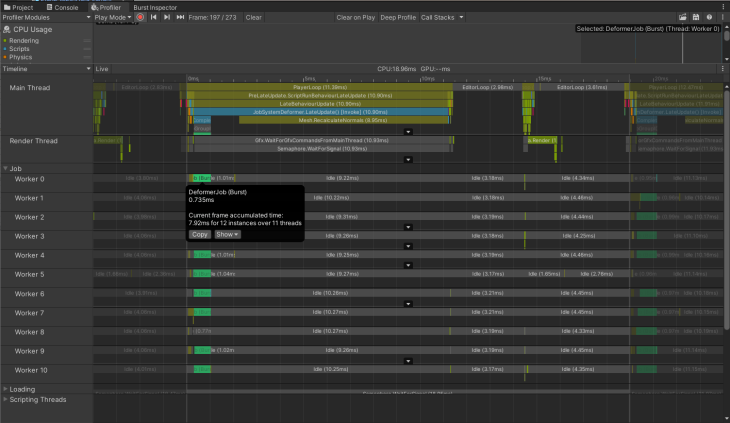### Mesh deformation with MeshData implementation

MeshData is a relatively new API that was added to Unity v2020.1. It provides a way to work with meshes within jobs, which allows us to get rid of the data buffer `NativeArray<Vector3> _vertices`.

This buffer was required because `mesh.vertices` return a copy of an actual array, so it was reasonable to cache this data and reuse the collection.

Instead, MeshData returns a pointer to the actual mesh data. From here, we can update the mesh every frame and get the pointer to the new data next frame before scheduling a new job — with no performance penalties related to allocating and copying large arrays.

So, the previous code transforms into:

```[BurstCompile]
public struct DeformMeshDataJob : IJobParallelFor
{
public Mesh.MeshData OutputMesh;

public DeformMeshDataJob(
NativeArray<VertexData> vertexData,
Mesh.MeshData outputMesh,
float speed,
float amplitude,
float time)
{
_vertexData = vertexData;
OutputMesh = outputMesh;
_speed = speed;
_amplitude = amplitude;
_time = time;
}

public void Execute(int index)
{
var outputVertexData = OutputMesh.GetVertexData<VertexData>();
var vertexData = _vertexData[index];
var position = vertexData.Position;
position.y = DeformerUtilities.CalculateDisplacement(position, _time, _speed, _amplitude);
outputVertexData[index] = new VertexData
{
Position = position,
Normal = vertexData.Normal,
Uv = vertexData.Uv
};
}
}
```

Here is how we get all the data needed to schedule the job:

```private void ScheduleJob()
{
...
// Will be writing into this mesh data
_meshDataArrayOutput = Mesh.AllocateWritableMeshData(1);
var outputMesh = _meshDataArrayOutput;
// From this one
var meshData = _meshDataArray;
// Set output mesh params
outputMesh.SetIndexBufferParams(meshData.GetSubMesh(0).indexCount, meshData.indexFormat);
outputMesh.SetVertexBufferParams(meshData.vertexCount, _layout);
// Get the pointer to the input vertex data array
_vertexData = meshData.GetVertexData<VertexData>();
_job = new DeformMeshDataJob(
_vertexData,
outputMesh,
_speed,
_amplitude,
Time.time
);

_jobHandle = _job.Schedule(meshData.vertexCount, _innerloopBatchCount);
}
```

You may have noticed that we get `meshData.GetVertexData<VertexData>()`, instead of just the vertices array:

```[StructLayout(LayoutKind.Sequential)]
public struct VertexData
{
public Vector3 Position;
public Vector3 Normal;
public Vector2 Uv;
}
```

This lets us set all the vertex data in the output mesh data inside the job. Contrary to the prior techniques, where we modify the mesh directly and the vertex data is already there, the output mesh data doesn’t contain the vertex data inside the job when created. When using this structure, make sure that it contains all the data your mesh has; otherwise, calling `GetVertexData<T>()` may fail or produce unwanted results.

For example, this one will work because it matches all vertex parameters: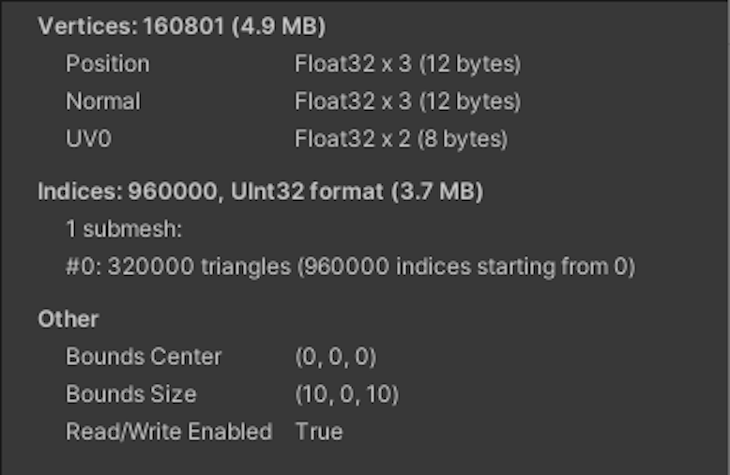However, this example will fail because of a tangent: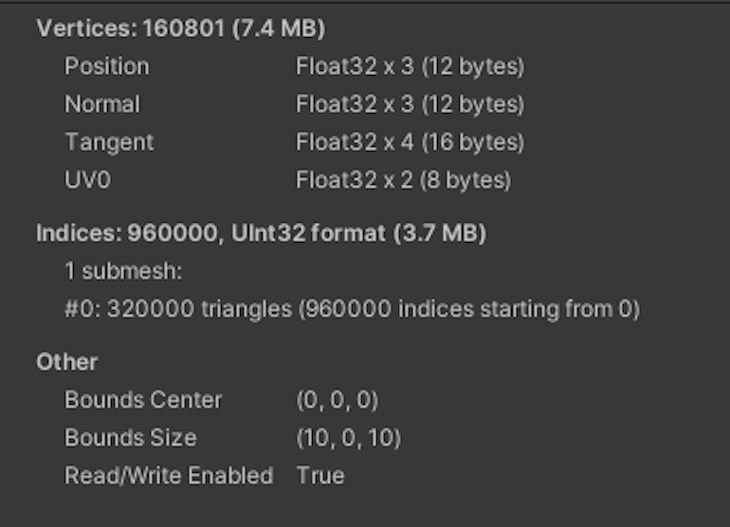If you need tangents, then you have to extend `VertexData` with the additional field. The same goes for any property you might add to a mesh.

After completing the job, we apply the data in the following way:

```private void UpdateMesh(Mesh.MeshData meshData)
{
// Get a reference to the index data and fill it from the input mesh data
var outputIndexData = meshData.GetIndexData<ushort>();
_meshDataArray.GetIndexData<ushort>().CopyTo(outputIndexData);
// According to docs calling Mesh.AcquireReadOnlyMeshData
// does not cause any memory allocations or data copies by default, as long as you dispose of the MeshDataArray before modifying the Mesh
_meshDataArray.Dispose();
meshData.subMeshCount = 1;
meshData.SetSubMesh(0,
_subMeshDescriptor,
MeshUpdateFlags.DontRecalculateBounds |
MeshUpdateFlags.DontValidateIndices |
MeshUpdateFlags.DontResetBoneBounds |
MeshUpdateFlags.DontNotifyMeshUsers);
Mesh.MarkDynamic();
Mesh.ApplyAndDisposeWritableMeshData(
_meshDataArrayOutput,
Mesh,
MeshUpdateFlags.DontRecalculateBounds |
MeshUpdateFlags.DontValidateIndices |
MeshUpdateFlags.DontResetBoneBounds |
MeshUpdateFlags.DontNotifyMeshUsers);
Mesh.RecalculateNormals();
}
```

Here is the entire script:

```public class MeshDataDeformer : BaseDeformer
{
private Vector3 _positionToDeform;
private Mesh.MeshDataArray _meshDataArray;
private Mesh.MeshDataArray _meshDataArrayOutput;
private VertexAttributeDescriptor[] _layout;
private SubMeshDescriptor _subMeshDescriptor;
private DeformMeshDataJob _job;
private JobHandle _jobHandle;
private bool _scheduled;

protected override void Awake()
{
base.Awake();
CreateMeshData();
}

private void CreateMeshData()
{
_layout = new[]
{
new VertexAttributeDescriptor(VertexAttribute.Position,
_meshDataArray.GetVertexAttributeFormat(VertexAttribute.Position), 3),
new VertexAttributeDescriptor(VertexAttribute.Normal,
_meshDataArray.GetVertexAttributeFormat(VertexAttribute.Normal), 3),
new VertexAttributeDescriptor(VertexAttribute.TexCoord0,
_meshDataArray.GetVertexAttributeFormat(VertexAttribute.TexCoord0), 2),
};
_subMeshDescriptor =
new SubMeshDescriptor(0, _meshDataArray.GetSubMesh(0).indexCount, MeshTopology.Triangles)
{
firstVertex = 0, vertexCount = _meshDataArray.vertexCount
};
}

private void Update()
{
ScheduleJob();
}

private void LateUpdate()
{
CompleteJob();
}

private void ScheduleJob()
{
if (_scheduled)
{
return;
}

_scheduled = true;
_meshDataArrayOutput = Mesh.AllocateWritableMeshData(1);
var outputMesh = _meshDataArrayOutput;
var meshData = _meshDataArray;
outputMesh.SetIndexBufferParams(meshData.GetSubMesh(0).indexCount, meshData.indexFormat);
outputMesh.SetVertexBufferParams(meshData.vertexCount, _layout);
_job = new DeformMeshDataJob(
meshData.GetVertexData<VertexData>(),
outputMesh,
_speed,
_amplitude,
Time.time
);

_jobHandle = _job.Schedule(meshData.vertexCount, 64);
}

private void CompleteJob()
{
if (!_scheduled)
{
return;
}

_jobHandle.Complete();
UpdateMesh(_job.OutputMesh);
_scheduled = false;
}

private void UpdateMesh(Mesh.MeshData meshData)
{
var outputIndexData = meshData.GetIndexData<ushort>();
_meshDataArray.GetIndexData<ushort>().CopyTo(outputIndexData);
_meshDataArray.Dispose();
meshData.subMeshCount = 1;
meshData.SetSubMesh(0,
_subMeshDescriptor,
MeshUpdateFlags.DontRecalculateBounds |
MeshUpdateFlags.DontValidateIndices |
MeshUpdateFlags.DontResetBoneBounds |
MeshUpdateFlags.DontNotifyMeshUsers);
Mesh.MarkDynamic();
Mesh.ApplyAndDisposeWritableMeshData(
_meshDataArrayOutput,
Mesh,
MeshUpdateFlags.DontRecalculateBounds |
MeshUpdateFlags.DontValidateIndices |
MeshUpdateFlags.DontResetBoneBounds |
MeshUpdateFlags.DontNotifyMeshUsers);
Mesh.RecalculateNormals();
}
}
```

### Deforming mesh with the compute shader implementation

Splitting the work over worker threads like in the previous example is a great idea, but we can split the work even more by offloading it to the GPU, which is designed to perform parallel work.

Here, the workflow is similar to the job system approach — we need to schedule a piece of work, but instead of using a job, we are going to use a compute shader and send the data to the GPU.

First, create a shader that uses `RWStructuredBuffer<VertexData>` as the data buffer instead of `NativeArray`. Apart from that and the syntax, the code is similar:

```#pragma kernel CSMain

struct VertexData
{
float3 position;
float3 normal;
float2 uv;
};

RWStructuredBuffer<VertexData> _VertexBuffer;
float _Time;
float _Speed;
float _Amplitude;

{
float3 position = _VertexBuffer[id.x].position;
const float distance = 6.0 - length(position - float3(0, 0, 0));
position.y = sin(_Time * _Speed + distance) * _Amplitude;
_VertexBuffer[id.x].position.y = position.y;
}
```

Pay attention to `VertexData`, which is defined at the top of the shader. We need its representation on the C# side too:

```[StructLayout(LayoutKind.Sequential)]
public struct VertexData
{
public Vector3 Position;
public Vector3 Normal;
public Vector2 Uv;
}
```

Here, we create the request `_request = AsyncGPUReadback.Request(_computeBuffer);` in `Update()` and collect the result if it’s ready in `LateUpdate()`:

```public class ComputeShaderDeformer : BaseDeformer
{
private bool _isDispatched;
private int _kernel;
private int _dispatchCount;
private ComputeBuffer _computeBuffer;

private NativeArray<VertexData> _vertexData;

// Cache property id to prevent Unity hashing it every frame under the hood

protected override void Awake()
{
{
gameObject.SetActive(false);
return;
}

base.Awake();
CreateVertexData();
SetMeshVertexBufferParams();
_computeBuffer = CreateComputeBuffer();
}

private void CreateVertexData()
{
// Can use here MeshData to fill the data buffer really fast and without generating garbage
}

private void SetMeshVertexBufferParams()
{
var layout = new[]
{
new VertexAttributeDescriptor(VertexAttribute.Position,
Mesh.GetVertexAttributeFormat(VertexAttribute.Position), 3),
new VertexAttributeDescriptor(VertexAttribute.Normal,
Mesh.GetVertexAttributeFormat(VertexAttribute.Normal), 3),
new VertexAttributeDescriptor(VertexAttribute.TexCoord0,
Mesh.GetVertexAttributeFormat(VertexAttribute.TexCoord0), 2),
};
Mesh.SetVertexBufferParams(Mesh.vertexCount, layout);
}

{
// No need to cache these properties to ids, as they are used only once and we can avoid odd memory usage
_dispatchCount = Mathf.CeilToInt(Mesh.vertexCount / threadX + 1);
}

private ComputeBuffer CreateComputeBuffer()
{
// 32 is the size of one element in the buffer. Has to match size of buffer type in the shader
// Vector3 + Vector3 + Vector2 = 8 floats = 8 * 4 bytes
var computeBuffer = new ComputeBuffer(Mesh.vertexCount, 32);
computeBuffer.SetData(_vertexData);
return computeBuffer;
}

private void Update()
{
Request();
}

private void LateUpdate()
{
TryGetResult();
}

private void Request()
{
if (_isDispatched)
{
return;
}

_isDispatched = true;
}

private void TryGetResult()
{
if (!_isDispatched || !_request.done)
{
return;
}

_isDispatched = false;
if (_request.hasError)
{
return;
}

_vertexData = _request.GetData<VertexData>();
Mesh.MarkDynamic();
Mesh.SetVertexBufferData(_vertexData, 0, 0, _vertexData.Length);
Mesh.RecalculateNormals();
}

private void OnDestroy()
{
_computeBuffer?.Release();
_vertexData.Dispose();
}
}
```

### Using Vertex Shader implementation in Unity

All previous techniques used different methods to modify data on the main or worker threads and on the GPU. Eventually, the data was passed back to the main thread to update `MeshFilter`.

Sometimes, you’ll need to update the `MeshCollider` so that Physics and Rigidbodies will work with your modified mesh. But what if there is no need to modify the Collider?

Imagine that you only need a visual effect using mesh deformation, such as leaves swaying in the wind. You can’t add many trees if every leaf is going to take part in physics calculations in every frame.

Luckily, we can modify and render a mesh without passing the data back to the CPU. To do that, we will add a displacement function to the Vertex Shader. This is a game-changer for performance because passing a large amount of data usually becomes a bottleneck in your game.

Of course, one buffer with mesh data will not make a significant difference. However, as your game expands, you should always profile to ensure that it won’t end up passing tons of different data for different features to the GPU and eventually become a bottleneck.

Next, we’ll add our properties to the `Properties` block:

```[PowerSlider(5.0)] _Speed ("Speed", Range (0.01, 100)) = 2
[PowerSlider(5.0)] _Amplitude ("Amplitude", Range (0.01, 5)) = 0.25
```

By default, there is no Vertex Shader function in the surface shader. We need to add `vertex:vert` to the surface definition:

```#pragma surface surf Standard fullforwardshadows vertex:vert addshadow
```

Additionally, `addshadow` is required for the surface shader to generate a shadow pass for new vertices’ positions instead of the original ones.

Now, we can define the `vert` function:

```SubShader
{
...
float _Speed;
float _Amplitude;

void vert(inout appdata_full data)
{
float4 position = data.vertex;
const float distance = 6.0 - length(data.vertex - float4(0, 0, 0, 0));
position.y += sin(_Time * _Speed + distance) * _Amplitude;
data.vertex = position;
}
...
}
```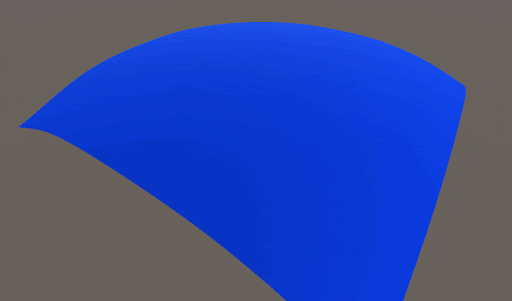That’s it! However, you might notice that because we don’t use `MonoBehaviour`, there’s nowhere to call `RecalculateNormals()`, and the deformation looks dull. Even if we had a separate component, calling `RecalculateNormals()` wouldn’t help because deformation only occurs on the GPU — meaning that no vertices data is passed back to the CPU. So, we’ll need to do it.

To do this, we can use the normal, tangent, and bitangent vectors. These vectors are orthogonal to each other. Because normal and tangent are in the data, we calculate the third one as `bitangent = cross(normal, tangent)`.

Given that they are orthogonal and that `normal` is perpendicular to the surface, we can find two neighboring points by adding `tangent` and `bitangent` to the current position.

```float3 posPlusTangent = data.vertex + data.tangent * _TangentMultiplier;
float3 posPlusBitangent = data.vertex + bitangent * _TangentMultiplier;
```

Don’t forget to use a small multiplier so that points are near the current vertex. Next, modify these vectors using the same displacement function:

```float getOffset( float3 position)
{
const float distance = 6.0 - length(position - float4(0, 0, 0, 0));
return sin(_Time * _Speed + distance) * _Amplitude;
}

void vert(inout appdata_full data)
{
data.vertex.y = getOffset(data.vertex);
...
posPlusTangent.y = getOffset(posPlusTangent);
posPlusBitangent.y = getOffset(posPlusBitangent);
...
float3 modifiedTangent = posPlusTangent - data.vertex;
float3 modifiedBitangent = posPlusBitangent - data.vertex;
float3 modifiedNormal = cross(modifiedTangent, modifiedBitangent);
data.normal = normalize(modifiedNormal);
}
```

Now, we subtract the current vertex from modified positions to get a new tangent and bitangent.

In the end, we can use the cross product to find the modified normal.

```float3 modifiedTangent = posPlusTangent - data.vertex;
float3 modifiedBitangent = posPlusBitangent - data.vertex;
float3 modifiedNormal = cross(modifiedTangent, modifiedBitangent);
data.normal = normalize(modifiedNormal);
```

This method is an approximation, but it gives plausible enough results to use in plenty of cases.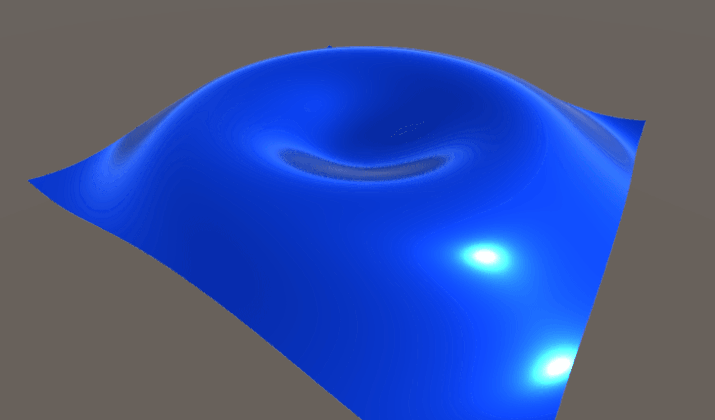Finally, here is the entire shader:

```Shader "Custom/DeformerSurfaceShader"
{
Properties
{
_Color ("Color", Color) = (1,1,1,1)
_MainTex ("Albedo (RGB)", 2D) = "white" {}
_Glossiness ("Smoothness", Range(0,1)) = 0.5
_Metallic ("Metallic", Range(0,1)) = 0.0
[PowerSlider(5.0)] _Speed ("Speed", Range (0.01, 100)) = 2
[PowerSlider(5.0)] _Amplitude ("Amplitude", Range (0.01, 5)) = 0.25
[PowerSlider(5.0)] _TangentMultiplier ("TangentMultiplier", Range (0.001, 2)) = 0.01
}
{
Tags
{
"RenderType"="Opaque"
}
LOD 200

CGPROGRAM

#pragma target 3.0

sampler2D _MainTex;

struct Input
{
float2 uv_MainTex;
};

half _Glossiness;
half _Metallic;
fixed4 _Color;
float _Speed;
float _Amplitude;
float _TangentMultiplier;

float getOffset( float3 position)
{
const float distance = 6.0 - length(position - float4(0, 0, 0, 0));
return sin(_Time * _Speed + distance) * _Amplitude;
}

void vert(inout appdata_full data)
{
data.vertex.y = getOffset(data.vertex);

float3 posPlusTangent = data.vertex + data.tangent * _TangentMultiplier;
posPlusTangent.y = getOffset(posPlusTangent);
float3 bitangent = cross(data.normal, data.tangent);

float3 posPlusBitangent = data.vertex + bitangent * _TangentMultiplier;
posPlusBitangent.y = getOffset(posPlusBitangent);

float3 modifiedTangent = posPlusTangent - data.vertex;
float3 modifiedBitangent = posPlusBitangent - data.vertex;
float3 modifiedNormal = cross(modifiedTangent, modifiedBitangent);
data.normal = normalize(modifiedNormal);
}

void surf(Input IN, inout SurfaceOutputStandard o)
{
fixed4 c = tex2D(_MainTex, IN.uv_MainTex) * _Color;
o.Albedo = c.rgb;
o.Metallic = _Metallic;
o.Smoothness = _Glossiness;
o.Alpha = c.a;
}
ENDCG
}
FallBack "Diffuse"
}
```

## Comparing performances of mesh deformation techniques in Unity

To compare all approaches, we are going to use the Performance Testing package by Unity.

Let’s look at a basic test that allows us to run the sample app for 500 frames. From there, we’ll gather frame times to see the median frame time for each technique that we’ve discussed. We can also use a simple `WaitForSeconds(x)` enumerator, but this will yield a different amount of samples in the run for each technique because frame times differ:

```[UnityTest, Performance]
public IEnumerator DeformableMeshPlane_MeshData_PerformanceTest()
{
yield return StartTest("Sample");
}

private static IEnumerator StartTest(string sceneName)
{
yield return RunTest();
}

{
yield return null;
}

private static IEnumerator RunTest()
{
var frameCount = 0;
using (Measure.Frames().Scope())
{
while (frameCount < 500)
{
frameCount++;
yield return null;
}
}
}
```

As an example, I ran the test suit on the following configuration:

```Intel Core i7-8750H CPU 2.20GHz (Coffee Lake), 1 CPU, 12 logical and 6 physical cores
NVIDIA GeForce GTX 1070
```

The mesh under `test` has 160,801 vertices and 320,000 triangles.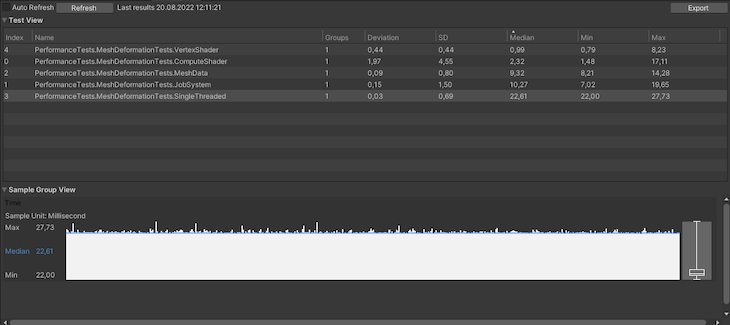## Conclusion

It’s clear that Vertex Shader is the winner here. However, it cannot cover all use cases that other techniques can, including updating a mesh Collider with the modified data.

Coming in second place is the compute shader, because it passes modified mesh data to the CPU, allowing it to be used in a wider variety of cases. Unfortunately, it is not supported on mobile before OpenGL ES v3.1.

The worst-performing approach we looked at was the single-threaded one. Although it is the simplest method, it can still fit into your frame budget if you have smaller meshes or are working on a prototype.

MeshData seems like a balanced approach if you’re targeting low-end mobile devices. You should check in the runtime if the current platform supports compute shaders, and then select MeshData or the compute shader deformer.

In the end, you must always profile your code before deciding about performance-critical parts of your game, so test your use case on a target platform when selecting any of these techniques. Check out the entire repository on GitHub.

# Get setup with LogRocket's modern error tracking in minutes:

1. Visit https://logrocket.com/signup/ to get an app ID.
2. Install LogRocket via NPM or script tag. `LogRocket.init()` must be called client-side, not server-side.
3. `\$ npm i --save logrocket // Code:import LogRocket from 'logrocket'; LogRocket.init('app/id');`
`Add to your HTML:<script src="https://cdn.lr-ingest.com/LogRocket.min.js"></script><script>window.LogRocket && window.LogRocket.init('app/id');</script>`
4. (Optional) Install plugins for deeper integrations with your stack:
• Redux middleware
• ngrx middleware
• Vuex pluginAlex Merzlikin Game developer with 8+ years of experience writing about different aspects of game development from performance and optimization to game architecture and clean code.